Problem

# The market for apple pies in the city of Ectenia is competitive and has the following de...

The market for apple pies in the city of Ectenia is competitive and has the following demand schedule: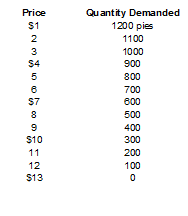Each procedure in the market has fixed costs of $9 and the following marginal cost: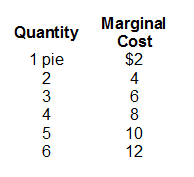a. Compute each procedure’s total cost and average total cost and for 1 to 6 pies. b. The price of a pie is now$11. How many pies are sold ? how many pies does each procedure make? How many producer are there? How much profit does each producer earn?

c. Is the situation described in part(b) a long-run equilibrium? Why or why not?

d. Suppose that in the long-run equilibrium? What is the market price and number of pies each procedure makes? How many pies are sold? How many pies producer are operating?

#### Step-by-Step Solution

Solution 1

There are n number of buyers and sellers, each selling homogenous good in a competitive market. Both buyers and sellers are price taker. At the equilibrium, marginal revenue, which is equal to price, must equal the marginal cost.

In the long run the firm exits the market if revenue it would get from producing less than its total cost. A firm may enter in the long run if the price of the good exceeds the average total cost of production.

(a)

Using the formula derives Total Cost (TC) and Average Cost (AC) is as follows: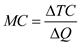Where,

Marginal Cost is represented by MC,

Change in Total Cost is represented by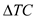and

Change in quantity is represented by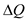.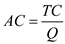Where,

Average Cost is represented by AC,

Total Cost is represented by TC, and

Quantity is represented by Q,

At zero level of quantity, there is no variable cost and hence total cost is equal to total fixed cost.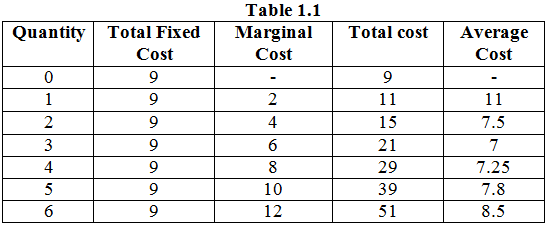(b)

With price equal to $$\ 11$$, the quantity demanded is 200 pies and the quantity supplied will be 5 pies as at the equilibrium each firm produces at the point where price equals marginal cost. There will be 40 sellers in the market, which is derived by diving the quantity demanded by the quantity supplied by each firm.

\begin{aligned} \text { Profit earned by each firm } &=\text { Total revenue }-\text { Total cost } \\ &=(\ 11 \times 5)-\ 39 \\ &=\ 55-\ 39 \\ &=\ 16 \end{aligned}

(c)

In the long run, price must equal the average total cost.

However, in this case the price is more than the average total cost of $$\ 7.8$$ which implies that there is opportunity for a firm to enter the market and earn positive profits.

(d)

In the long run, firms will enter the market until profit is driven to zero. Hence the price must be equal to the minimum of average total cost, which is $$\ 7$$.

In the long run equilibrium each firm earns normal profit. Each producer makes 3 pies at this level.

The number of pie producers in the market $$=\frac{600}{3}$$

$$=200$$